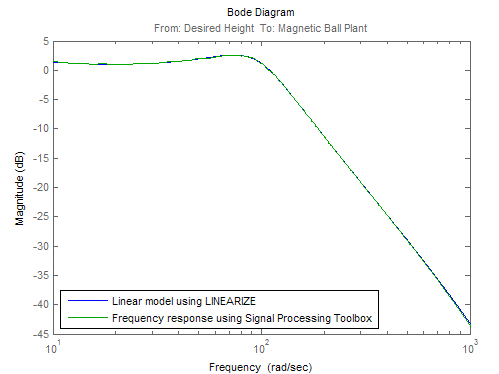Estimate Frequency Response Models with Noise Using Signal Processing Toolbox

Open the Simulink® model, and specify which portion of the model to linearize:

io(1) = linio('magball/Desired Height',1);
io(2) = linio('magball/Magnetic Ball Plant',1,'output');

Create a random input signal for simulation:

in = frest.Random('Ts',0.001,'NumSamples',1e4);

Linearize the model at a steady-state operating point:

op = findop('magball',operspec('magball'),...
findopOptions('DisplayReport','off'));
sys = linearize('magball',io,op);

Simulate the model to obtain the output at the linearization output point:

[sysest,simout] = frestimate('magball',io,in,op);

Estimate a frequency response model using Signal Processing Toolbox™ software, which includes windowing and averaging:

input = generateTimeseries(in);
output = detrend(simout{1}.Data,'constant');
[Txy,F] = tfestimate(input.Data(:),...
output,hanning(4000),[],4000,1/in.Ts);
systfest = frd(Txy,2*pi*F);

Compare the results of analytical linearization and tfestimate (Signal Processing Toolbox):

ax = axes;
h = bodeplot(ax,sys,'b',systfest,'g',systfest.Frequency);
setoptions(h,'Xlim',[10,1000],'PhaseVisible','off')
legend(ax,'Linear model using LINEARIZE','Frequency response using Signal Processing Toolbox',...
'Location','SouthWest')In this case, the Signal Processing Toolbox command tfestimate (Signal Processing Toolbox) gives a more accurate estimation than frestimate due to windowing and averaging.